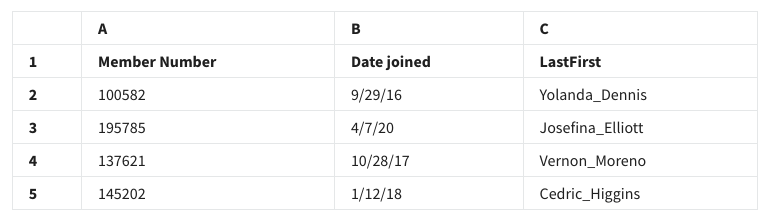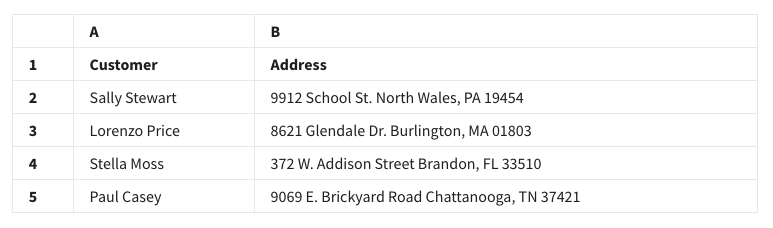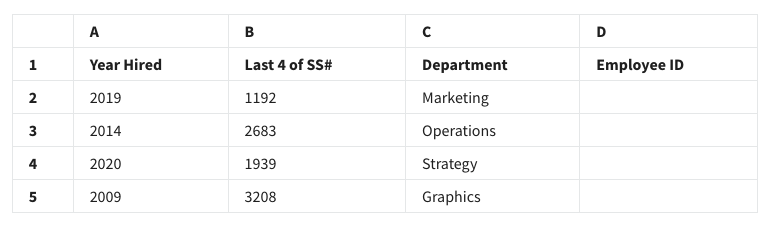# process data from dirty to clean weekly challenge 2 answers

• True
• False

#### 2.A data analyst at a nonprofit organization is working with the following spreadsheet, which contains member name data in column C. They want to divide this data using the underscore as a delimiter, so that first names are stored in one column and last names in another. Which tool should the analyst use?• Conditional formatting
• Pivot table
• SPLIT function
• MID function

• syntax
• standard
• structure
• script

#### 4.You are working with the following selection of a spreadsheet:• =RIGHT(B3,5)
• =RIGHT(5,B3)
• =LEFT(5,B3)
• =LEFT(B3,5)

#### 5. A data analyst in a human resources department is working with the following selection of a spreadsheet:#### They want to create employee identification numbers (IDs) in column D. The IDs should include the year hired plus the last four digits of the employee’s Social Security Number (SS#). What function will create the ID 20093208 for the employee in row 5?

• =CONCATENATE(A5!B5)
• =CONCATENATE(A5*B5)
• =CONCATENATE(A5+B5)
• =CONCATENATE(A5,B5)

• SUMIF
• COUNTIF
• COUNT
• SUM

• VALUE
• VLOOKUP
• MATCH
• FIND

#### 8. A data analyst needs to combine two datasets. Each dataset comes from a different system, and the systems store data in different ways. What can the data analyst do to ensure the data is compatible?

• Use a data visualization
• Map the data
• Apply a data structure
• Merge the data

## Shuffle Q/A 1

#### 9. In their spreadsheet, a data analyst makes cells stand out for more efficient analysis. What spreadsheet tool is used to do this?

• Cell filtering
• Conditional ranking
• Conditional formatting
• Cell querying

• Unit
• Delimiter
• Partition
• Substring